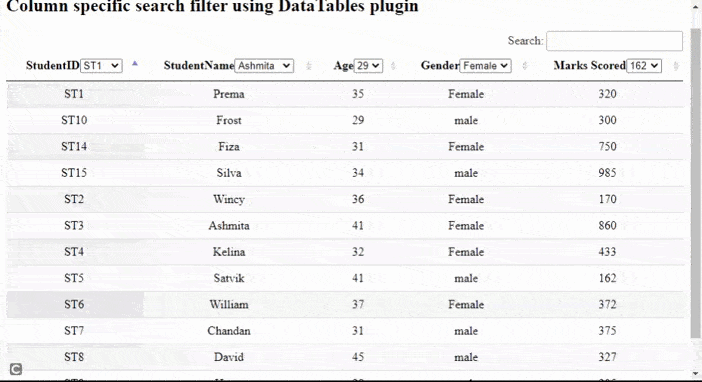# How to implement column specific search filter using DataTables plugin ?

• Difficulty Level : Medium
• Last Updated : 11 Nov, 2021

DataTables are a modern jQuery plugin for adding interactive and advanced controls to HTML tables for a webpage. It is a very simple-to-use plug-in with a variety of options for the developer’s custom changes as per the application’s need. The plugin’s features include pagination, sorting, searching, and multiple-column ordering.

In this article, we will demonstrate the implementation of a column-specific search filter using the DataTables plugin. Instead of performing search operations on the whole table, the search is performed only on a particular column for improving the performance of the system

Approach: In the following example, DataTables uses the student details from the HTML table as the main source. Each row in the table shows details for one student’s information.

• A DataTable is initialized.
• The developer can set the features of paging or searching as per the need as shown in the script part of the code.
• The column() API is used to select all the columns of the table.
• The flatten() API is used to convert the 2D array structure into a single dimension array and each() method is used to perform any action on each of the selected columns.
• The select list is appended to each column header.
• Any action is performed on change of any list value.
• The column().search() API is used to search the selected value and the draw() API is used to reflect the changes after the action in the table.
• column().cache() API is used to get the data from the column with sort() method to display sorted data.
• All the steps are done for each of the columns.

The pre-compiled files which are needed to implement are

CSS CDN:

`https://cdn.datatables.net/1.10.22/css/jquery.dataTables.min.css`

JavaScript CDN:

```https://code.jquery.com/jquery-3.5.1.js
https://cdn.datatables.net/1.10.22/js/jquery.dataTables.min.js```

Example:  The following example demonstrates the above approach showing column-specific search filter operation.

## HTML

 `` `<``html``>`   `<``head``>` `  ``<``meta` `content="``initial-scale``=``1``, ` `         ``maximum-scale``=``1``, ``user-scalable``=``0``" ``name``=``"viewport"` `/>` `  ``<``meta` `name``=``"viewport"` `content``=``"width=device-width"` `/>`   `  ``` `  ``<``link` `rel``=``"stylesheet"` `href``=` `"https://cdn.datatables.net/1.10.22/css/jquery.dataTables.min.css"` `/>` `  ``` `  ``<``script` `type``=``"text/javascript"` `src``=` `    ``"https://code.jquery.com/jquery-3.5.1.js"``>` `  ``` `  `  `  ``` `  ``<``script` `type``=``"text/javascript"` `src``=` `"https://cdn.datatables.net/1.10.22/js/jquery.dataTables.min.js"``>` `  ```   `  ``<``style``>` `    ``td {` `      ``text-align: center;` `    ``}` `  ``` ``   `<``body``>` `  ``<``h2``>` `    ``Column specific search filter` `    ``using DataTables plugin` `  ```   `  ``` `  ``<``table` `id``=``"tableID"` `class``=``"display"` `      ``style``=``"width: 100%"``>` `    ``<``thead``>` `      ``<``tr``>` `        ``<``th``>StudentID` `        ``<``th``>StudentName` `        ``<``th``>Age` `        ``<``th``>Gender` `        ``<``th``>Marks Scored` `      ``` `    ``` `    ``<``tbody``>` `      ``<``tr``>` `        ``<``td``>ST1` `        ``<``td``>Prema` `        ``<``td``>35` `        ``<``td``>Female` `        ``<``td``>320` `      ``` `      ``<``tr``>` `        ``<``td``>ST2` `        ``<``td``>Wincy` `        ``<``td``>36` `        ``<``td``>Female` `        ``<``td``>170` `      ``` `      ``<``tr``>` `        ``<``td``>ST3` `        ``<``td``>Ashmita`   `        ``<``td``>41` `        ``<``td``>Female` `        ``<``td``>860` `      ``` `      ``<``tr``>` `        ``<``td``>ST4` `        ``<``td``>Kelina` `        ``<``td``>32` `        ``<``td``>Female` `        ``<``td``>433` `      ``` `      ``<``tr``>` `        ``<``td``>ST5` `        ``<``td``>Satvik` `        ``<``td``>41` `        ``<``td``>male` `        ``<``td``>162` `      ``` `      ``<``tr``>` `        ``<``td``>ST6` `        ``<``td``>William` `        ``<``td``>37` `        ``<``td``>Female` `        ``<``td``>372` `      ``` `      ``<``tr``>` `        ``<``td``>ST7` `        ``<``td``>Chandan` `        ``<``td``>31` `        ``<``td``>male` `        ``<``td``>375` `      ``` `      ``<``tr``>` `        ``<``td``>ST8` `        ``<``td``>David` `        ``<``td``>45` `        ``<``td``>male` `        ``<``td``>327` `      ``` `      ``<``tr``>` `        ``<``td``>ST9` `        ``<``td``>Harry` `        ``<``td``>29` `        ``<``td``>male` `        ``<``td``>205` `      ``` `      ``<``tr``>` `        ``<``td``>ST10` `        ``<``td``>Frost` `        ``<``td``>29` `        ``<``td``>male` `        ``<``td``>300` `      ```   `      ``<``tr``>` `        ``<``td``>ST14` `        ``<``td``>Fiza` `        ``<``td``>31` `        ``<``td``>Female` `        ``<``td``>750` `      ``` `      ``<``tr``>` `        ``<``td``>ST15` `        ``<``td``>Silva` `        ``<``td``>34` `        ``<``td``>male` `        ``<``td``>985` `      ``` `    ``` `  ``` `  ``<``br` `/>`   `  ``<``script``>`   `      ``/* Initialization of datatables */` `      ``\$(document).ready(function () {`   `        ``// Paging and other information are` `        ``// disabled if required, set to true` `        ``var myTable = \$("#tableID").DataTable({` `          ``paging: false,` `          ``searching: true,` `          ``info: false,` `        ``});`   `        ``// 2d array is converted to 1D array` `        ``// structure the actions are ` `        ``// implemented on EACH column` `        ``myTable` `          ``.columns()` `          ``.flatten()` `          ``.each(function (colID) {`   `            ``// Create the select list in the` `            ``// header column header` `            ``// On change of the list values,` `            ``// perform search operation` `            ``var mySelectList = \$("<``select` `/>")` `              ``.appendTo(myTable.column(colID).header())` `              ``.on("change", function () {` `                ``myTable.column(colID).search(\$(this).val());`   `                ``// update the changes using draw() method` `                ``myTable.column(colID).draw();` `              ``});`   `            ``// Get the search cached data for the` `            ``// first column add to the select list` `            ``// using append() method` `            ``// sort the data to display to user` `            ``// all steps are done for EACH column` `            ``myTable` `              ``.column(colID)` `              ``.cache("search")` `              ``.sort()` `              ``.each(function (param) {` `                ``mySelectList.append(` `                  ``\$('<``option` `value``=``"' + param + '"``>'` `                    ``+ param + "")` `                ``);` `              ``});` `          ``});` `      ``});` `  ``` ``   ``

Output:My Personal Notes arrow_drop_up
Recommended Articles
Page :